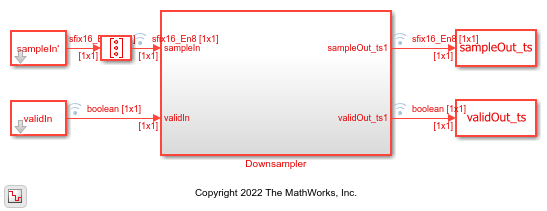# Implement Downsampler For HDL

This example shows how to use the Downsampler block to downsample data. This block supports scalar and vector inputs. You can generate HDL code from the subsystem used in this example.

### Set Up Input Data Parameters

Set up workspace variables for the Simulink® model to use. These variables configure the Downsampler block inside the subsystem.

```K = 8; % Downsample factor O = 3; % Sample offset scalar = true; if scalar vecSize = 1; value = 1; else vecSize = 16; %#ok % Vector size must be a multiple or factor of K value = vecSize/K; end ```

### Generate Reference Data From Function

Generate frames of random input samples and apply to the `downsample` function. Use the output as reference data against which to compare the output of the block.

```totalsamples = 0; numFrames = 1; dataSamples = cell(1,numFrames); framesize = zeros(1,numFrames); refOutput = []; WL = 0; % Word length FL = 0; % Fraction length for i = 1:numFrames framesize(i) = randi([5 200],1,1)*vecSize; dataSamples{i} = fi(randn(vecSize,framesize(i)),1,16,8); ref_downsample= downsample((dataSamples{i}(:)),K,O); refOutput = [refOutput,ref_downsample]; %#ok end ```

### Run Model

Run the model to import the input signal variables from the MATLAB® workspace to the Downsampler block in the model. Insert the required number of idle cycles after each frame using the `latency` variable to avoid invalid output data. Initialize the size of the output to accommodate the output data.

```latency = 2+O; sampleIn = dataSamples{i}; validIn = true(1,sum(framesize)); sampletime = 1; simTime = length(validIn)+latency; modelname = "HDLDownsampler"; open_system(modelname); sim(modelname); ```### Compare Block Output with Function Output

Compare the output of the block with the output of the `Downsampler` function.

```sampleOut = squeeze(sampleOut_ts.Data); validOut = squeeze(validOut_ts.Data); if scalar HDLOutput = sampleOut(validOut); else HDLOutput = sampleOut(:,validOut); %#ok end HDLOutput = HDLOutput(:); fprintf('\n Downsampler\n'); difference = (abs(HDLOutput-refOutput(1:length(HDLOutput)))>0); fprintf(['\nTotal number of samples differed between Behavioral ' ... 'and HDL simulation: %d \n'],sum(difference)); ```
``` Downsampler Total number of samples differed between Behavioral and HDL simulation: 0 ```